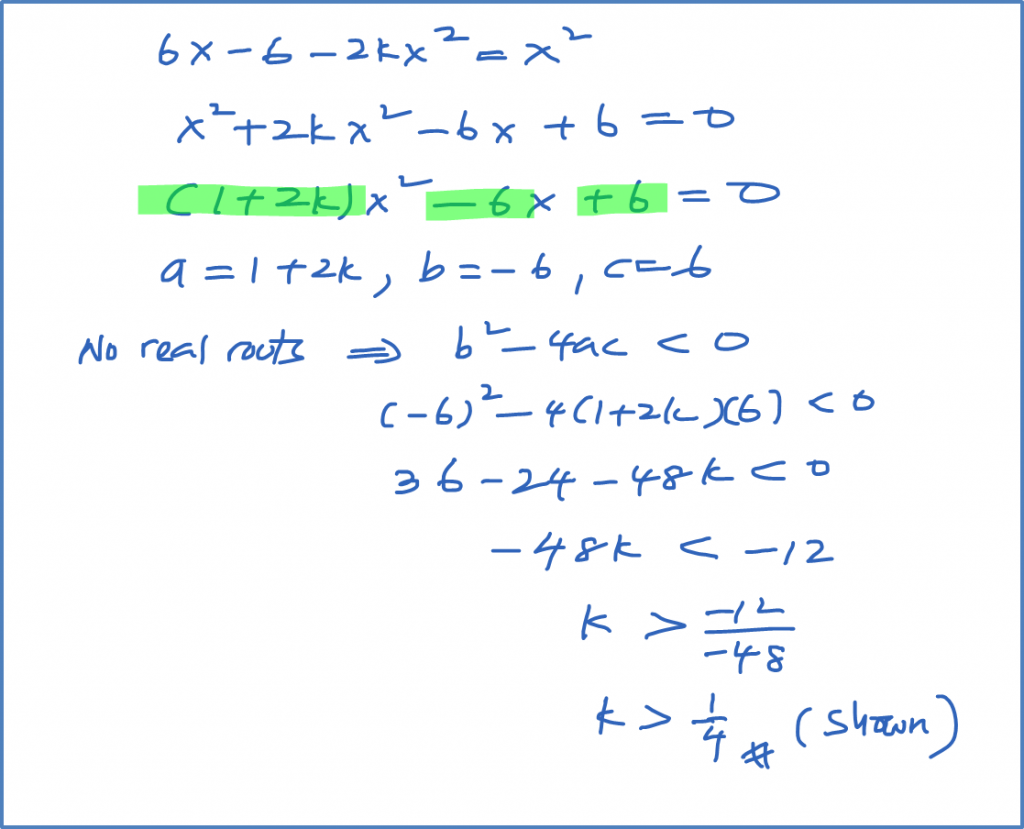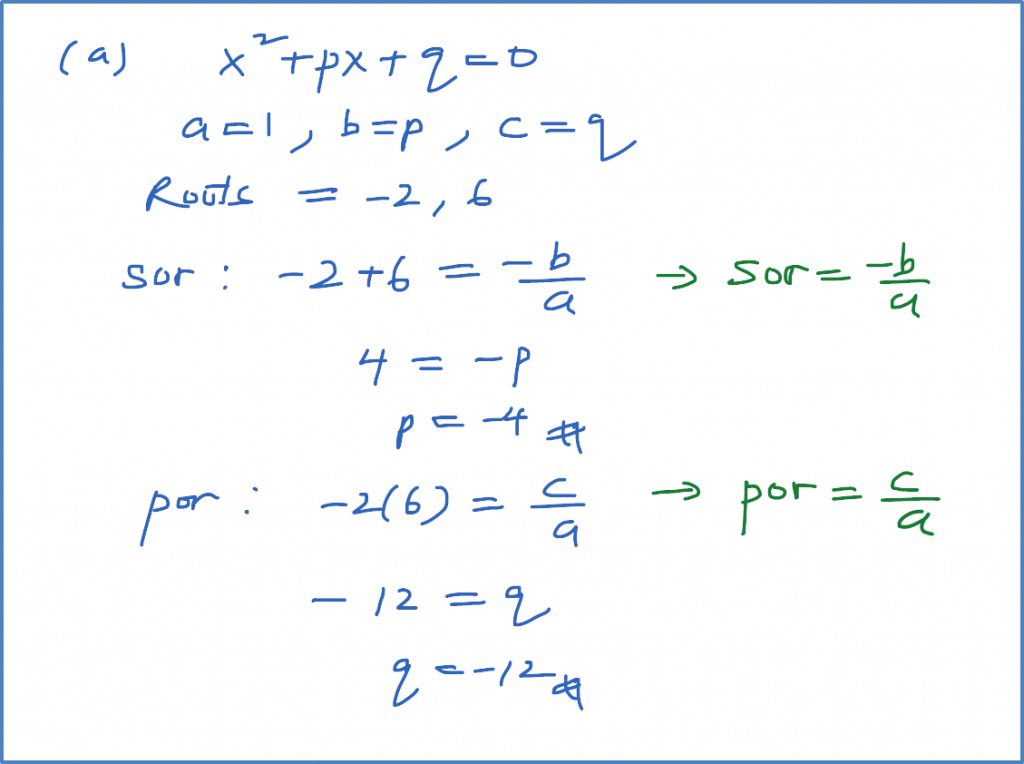\

# 2.12.5 Quadratic Functions, SPM Practice (Paper 1)

Question 13:

Solution:Question 14:
The quadratic equation ${x}^{2}+px+q=0$ has roots –2 and 6. Find
(a) the value of p and of q,
(b) the range of values of r for which the equation ${x}^{2}+px+q=r$ has no real roots.

Solution:Question 15:
The straight line y = 5x – 1 does not intersect with the curve y = 2x2 + x + h.
Find the range of values of h.

Solution:

Question 16:
Find the range of values of p for which the equation $2{x}^{2}+5x+3-p=0$ has two real distinct roots.

Solution: